×#### Thank you for registering.

One of our academic counsellors will contact you within 1 working day.

Click to Chat

1800-1023-196

+91-120-4616500

CART 0

• 0

MY CART (5)

Use Coupon: CART20 and get 20% off on all online Study Material

ITEM
DETAILS
MRP
DISCOUNT
FINAL PRICE
Total Price: Rs.

There are no items in this cart.
Continue Shopping• Complete JEE Main/Advanced Course and Test Series
• OFFERED PRICE: Rs. 15,900
• View Details

```Chapter 12: Heron’s Formula Exercise – 12.2

Question: 1

Find the area of the quadrilateral ABCD in which AB = 3 cm, BC = 4 cm, CD = 4 cm, DA = 5 cm and AC = 5 cm.

Solution:

For triangle ABC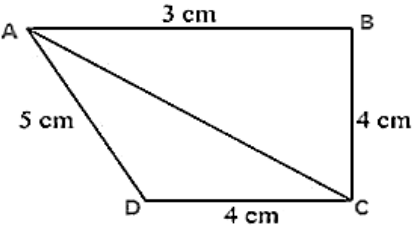AC2 = BC2 + AB2

25 = 9 + 16

So, triangle ABC is a right angle triangle right angled at point R

Area of triangle ABC = 12 × AB × BC

= 1/2 × 3 × 4

= 6 cm2

Perimeter = 2s = AC + CD + DA

2s = 5 cm+ 4 cm+ 5 cm

2s = 14 cm

s = 7 cm

By using Heron's Formula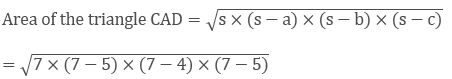= 9.16 cm2

Area of ABCD = Area of ABC + Area of CAD

= (6 + 9.16) cm2

= 15.16 cm2

Question: 2

The sides of a quadrilateral field, taken in order are 26 m, 27 m, 7 m, 24 m respectively. The angle contained by the last two sides is a right angle. Find its area.

Solution:

Here the length of the sides of the quadrilateral is given asAB = 26 m, BC = 27 m, CD = 7 m, DA = 24 m

Diagonal AC is joined.

By applying Pythagoras theorem

AC2 = 142  + 72

AC = 25 m

Now area of triangle ABC

Perimeter = 2s = AB + BC + CA

2s = 26 m + 27 m + 25 m

s = 39 m

By using Heron's Formula= 291.84 m2

Thus, the area of a triangle is 291.84 m2

Now for area of triangle ADC

Perimeter = 2S = AD + CD + AC

= 25 m + 24 m + 7 m

S = 28 m

By using Heron's Formula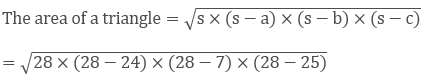= 84 m2

Thus, the area of a triangle is 84m2

Therefore, Area of rectangular field ABCD

= Area of triangle ABC + Area of triangle ADC

= 291.84 + 84

= 375.8 m2

Question: 3

The sides of a quadrilateral, taken in order as 5 m, 12 m, 14 m, 15 m respectively, and the angle contained by first two sides is a right angle. Find its area.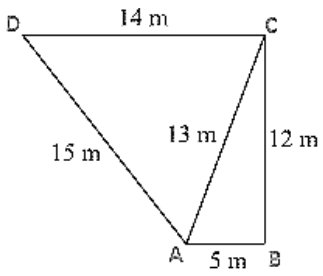Solution:

Given that the sides of the quadrilateral are

AB = 5 m, BC = 12 m, CD =14 m and DA = 15 m

Join AC

Now area of triangle ABC = ½ × AB × BC

= 1/2× 5 × 12

= 30 m2

In triangle ABC, By applying Pythagoras theorem

AC2 = AB2 + BC2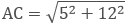AC = 13 m

Perimeter = 2s = AD + DC + AC

2s = 15 m + 14 m + 13 m

s = 21 m

By using Heron's Formula,= 84 m2

Area of quadrilateral ABCD = Area of triangle ABC + Area of triangle ADC

= (30 + 84) m2

= 114 m2

Question: 4

A park in the shape of a quadrilateral ABCD, has angle C = 90°, AB = 9 m, BC = 12 m, CD = 5 m, AD = 8 m. How much area does it occupy?

Solution:

Given sides of a quadrilateral are AB = 9 m, BC = 12 m, CD = 5 m, DA = 8 m.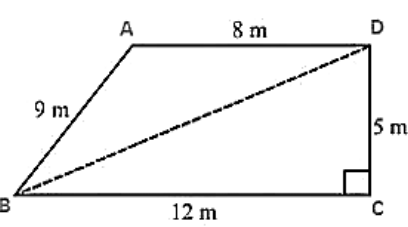Let us join BD

In triangle BCD, apply Pythagoras theorem

BD2 = BC2 + CD2

BD2 = 122 + 52

BD = 13 m

Area of triangle BCD = 1/2 × BC × CD

= 1/2 × 12 × 5

= 30 m2

Now, in triangle ABD

Perimeter = 2s = 9 m + 8m + 13m

s = 15 m

By using Heron's Formula,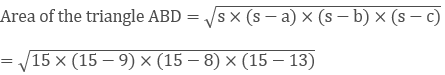= 35.49 m2

Area of quadrilateral ABCD = Area of triangle ABD + Area of triangle BCD

= (35.496 + 30) m2

= 65.5m2.

Question: 5

Two parallel sides of a trapezium are 60 m and 77 m and the other sides are 25 m and 26 m. Find the area of the trapezium?

Solution:

Given,

Two parallel sides of trapezium are AB = 77 m and CD = 60 m

The other two parallel sides of trapezium are BC = 26 m, AD = 25mJoin AE and CF

DE is perpendicular to AB and also, CF is perpendicular to AB

Therefore, DC = EF = 60 m

Let AE = x

So, BF = 77 - 60 - x

BF = 17 - x

By using Pythagoras theorem,

DE2 = 252 − x2

In triangle BCF,

By using Pythagoras theorem,

CF2 = BC2 − BF2

CF2 = 262 − (17 − x)2

Here, DE = CF

So, DE2 = CF2

252 − x2 = 262 − (17 − x)2

252 − x2 = 262 − (172 − 34x + x2)

252 − x2 = 262 − 172 + 34x + x2

252 = 262 − 172 + 34x

x = 7

DE2 = 252 − x2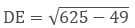DE = 24 m

Area of trapezium = 1/2 × (60 + 77) × 24

Area of trapezium = 1644 m2

Question: 6

Find the area of a rhombus whose perimeter is 80 m and one of whose diagonal is 24 m.

Solution:

Given,

Perimeter of a rhombus = 80 m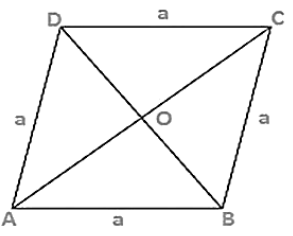As we know,

Perimeter of a rhombus = 4 × side = 4 × a

4 × a = 80 m

a = 20 m

Let AC = 24 m

Therefore OA = 1/2 × AC

OA = 12 m

In triangle AOB

OB2 = AB2 − OA2

OB2 = 202 − 122

OB = 16 m

Also, OB = OD because diagonal of rhombus bisect each other at 90°

Therefore, BD = 2 OB = 2 × 16 = 32 m

Area of rhombus = 1/2 × BD × AC

Area of rhombus = 1/2 × 32 × 24

Area of rhombus = 384 m2

Question: 7

A rhombus sheet, whose perimeter is 32 m and whose diagonal is 10 m long, is painted on both the sides at the rate of Rs 5 per meter square. Find the cost of painting.

Solution:

Given that,

Perimeter of a rhombus = 32 m

We know that,

Perimeter of a rhombus = 4 × side

4 × side = 32 m

4 × a = 32 m

a = 8 m

Let AC = 10 m

OA = 12 × AC

OA = 12 × 10

OA = 5 m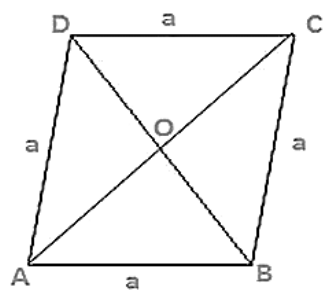By using Pythagoras theorem

OB2 = AB2 − OA2

OB2 = 82 − 52

OB = √39 m

BD = 2 × OB

BD = 2√39 m

Area of the sheet = 1/2 × BD × AC

Area of the sheet = 1/2 ×2√39 × 10

Therefore, cost of printing on both sides at the rate of Rs. 5 per m2

= Rs 2 × 10√39 × 5

= Rs. 625

Question: 8

Find the area of the quadrilateral ABCD in which AD = 24 cm, angle BAD = 90° and BCD forms an equilateral triangle whose each side is equal to 26 cm. [Take √3 = 1.73]

Solution:

Given that, in a quadrilateral ABCD in which AD = 24 cm,

BCD is an equilateral triangle and the sides BC = CD = BD = 26 cm

In triangle BAD, by applying Pythagoras theorem,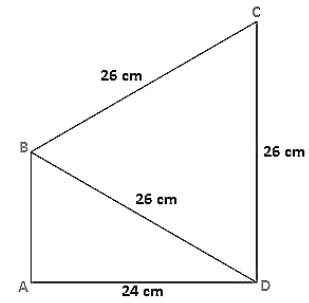BA2 = BD2 − AD2

BA2 = 262 + 242

BA = √100

BA = 10 cm

Area of the triangle BAD = 1/2 × 10 × 24

Area of the triangle BAD = 120 cm2

Area of the equilateral triangle = √3/4 × side

Area of the equilateral triangle QRS = √3/4 × 26

Area of the equilateral triangle BCD = 292.37 cm2

Therefore, the area of quadrilateral ABCD = Area of triangle BAD + Area of the triangle BCD

The area of quadrilateral ABCD = 120 + 292.37

= 412.37 cm2

Question: 9

Find the area of quadrilateral ABCD in which AB = 42 cm, BC = 21 cm, CD = 29 cm, DA = 34 cm and the diagonal BD = 20 cm.

Solution:

Given

AB = 42 cm, BC = 21 cm, CD = 29 cm, DA = 34 cm, and the diagonal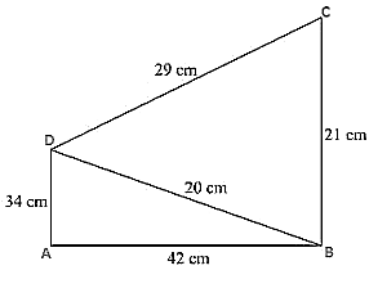BD = 20 cm.

Now, for the area of triangle ABD

Perimeter of triangle ABD 2s = AB + BD + DA

2s = 34 cm + 42 cm + 20 cm

s = 48 cm

By using Heron’s Formula,= 33 6cm2

Now, for the area of triangle BCD

Perimeter of triangle BCD 2s = BC + CD + BD

2s = 29cm + 21cm + 20cm

s = 35 cm

By using Heron's Formula,= 210 cm2

Therefore, Area of quadrilateral ABCD = Area of triangle ABD + Area of triangle BCD

Area of quadrilateral ABCD = 336 + 210

Area of quadrilateral ABCD = 546 cm2

Question: 10

Find the perimeter and the area of the quadrilateral ABCD in which AB = 17 cm, AD = 9 cm, CD = 12 cm, AC = 15 cm and angle ACB = 90°.

Solution:

Given are the sides of the quadrilateral ABCD in which

AB = 17 cm, AD = 9 cm, CD = 12 cm, AC = 15 cm and an angle ACB = 90°

By using Pythagoras theorem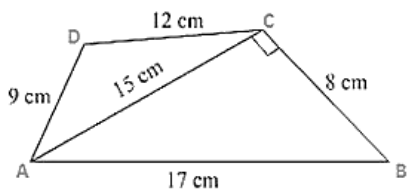BC2 = AB2 − AC2

BC2 = 172 − 152

BC = 8 cm

Now, area of triangle ABC = ½ × AC × BC

Area of triangle ABC = 1/2 × 8 × 15

Area of triangle ABC = 60 cm2

Now, for the area of triangle ACD

Perimeter of triangle ACD 2s = AC + CD + AD

2s = 15 + 12 + 9

s = 18 cm

By using Heron's Formula,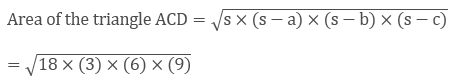= 54 cm2

Area of quadrilateral ABCD = Area of triangle ABC + Area of triangle ACD

Area of quadrilateral ABCD = 60 cm2 + 54 cm2

Area of quadrilateral ABCD = 114 cm2

Question: 11

The adjacent sides of a parallelogram ABCD measure 34 cm and 20 cm, and the diagonal AC measures 42 cm. Find the area of parallelogram.

Solution:

The adjacent sides of a parallelogram ABCD measures 34 cm and 20 cm, and the diagonal AC measures 42 cm.

Area of the parallelogram = Area of triangle ADC + Area of triangle ABC

Note: Diagonal of a parallelogram divides into two congruent trianglesTherefore,

Area of the parallelogram = 2 × (Area of triangle ABC)

Now, for area of triangle ABC

Perimeter = 2s = AB + BC + CA

2s = 34 cm + 20 cm + 42 cm

s = 48 cm

By using Heron's Formula,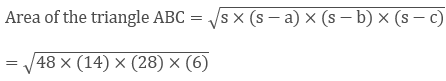= 336 cm2

Therefore, area of parallelogram ABCD = 2 × (Area of triangle ABC)

Area of parallelogram = 2 × 336 cm2

Area of parallelogram ABCD = 672 cm2

Question: 12

Find the area of the blades of the magnetic compass shown in figure given below:Solution:

Area of the blades of magnetic compass = Area of triangle ADB + Area of triangle CDB

Now, for the area of triangle ADB

Perimeter = 2s = AD + DB + BA

2s = 5 cm + 1 cm + 5 cm

s = 5.5 cm

By using Heron's Formula,= 2.49 cm2

Also, area of triangle ADB = Area of triangle CDB

Therefore area of the blades of the magnetic compass = 2 × area of triangle ADB

Area of the blades of the magnetic compass = 2 × 2.49

Area of the blades of the magnetic compass = 4.98 cm2

Question: 13

A hand fan is made by sticking 10 equal size triangular strips of two different types of paper as shown in the figure. The dimensions of equal strips are 25 cm, 25 cm and 14 cm. Find the area of each type of paper needed to make the hand fan.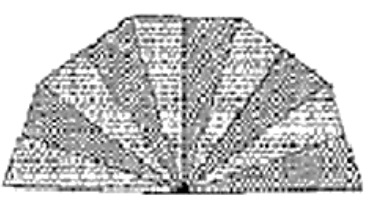Solution:

Given that,

The sides of AOB

AO = 25 cm

OB = 25 cm

BA = 14 cm

Area of each strip = Area of triangle AOB

Now, for the area of triangle AOB

Perimeter = AO + OB + BA

2s = 25 cm +25 cm + 14 cm

s = 32 cm

By using Heron's Formula,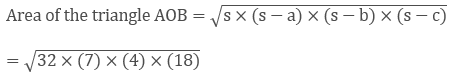= 168 cm2

Also, area of each type of paper needed to make a fan = 5 × Area of triangle AOB

Area of each type of paper needed to make a fan = 5 × 168 cm2

Area of each type of paper needed to make a fan = 840 cm2

Question: 14

A triangle and a parallelogram have the same base and the same area. If the sides of the triangle are 13 cm, 14 cm and 15 cm and the parallelogram stands on the base 14 cm, find the height of a parallelogram.

Solution:

The sides of the triangle DCE are

DC = 15 cm,

CE = 13 cm,

ED = 14 cm

Let the h be the height of parallelogram ABCD

Now, for the area of triangle DCE

Perimeter = DC + CE + ED

2s = 15 cm + 13 cm + 14 cm

s = 21 cm

By using Heron's Formula,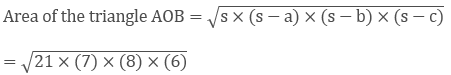= 84 cm2

Also, area of triangle DCE = Area of parallelogram ABCD ⟹ 84 cm2

24 × h = 84 cm2

h = 6 cm.
```### Course Features

• 728 Video Lectures
• Revision Notes
• Previous Year Papers
• Mind Map
• Study Planner
• NCERT Solutions
• Discussion Forum
• Test paper with Video Solution